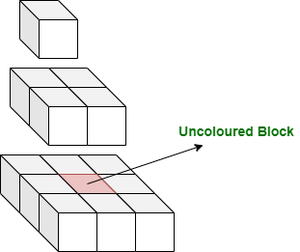Count of uncolored cells in a Pyramid of length H formed using cubes

• Last Updated : 12 Nov, 2021

Given a pyramid, formed using unit area cubes, of given height H. The pyramid is then placed on the ground and painted from outside. The task is to find the count of cubes that are left uncoloured.

Examples:

Input: H = 3
Output: 1
Explanation:Input: H = 1
Output: 0

Naive Approach: The number of cubes that will remain uncoloured is the number of cubes that are not present in the boundary of the pyramid. So, for every layer of cubes at height h, the number of uncoloured cubes iswhere h>1. So, add the number of uncoloured cubes at every level to get the answer.

Below is the implementation of the above approach:

C++

 // C++ program for the above problem #include using namespace std; // Function to return the number of// uncoloured cubesint uncolouredCubes(int H){     // To store the number of uncoloured    // cubes    int res = 0;     for (int h = 2; h <= H; h++) {        res += (h - 2) * (h - 2);    }     return res;} // Driver Codeint main(){    int H = 3;    cout << uncolouredCubes(H);}

Java

 // Java program for the above problem  class GFG{   // Function to return the number of  // uncoloured cubes  static int uncolouredCubes(int H)  {       // To store the number of uncoloured      // cubes      int res = 0;       for (int h = 2; h <= H; h++) {          res += (h - 2) * (h - 2);      }       return res;  }   // Driver Code  public static void main(String [] args)  {      int H = 3;      System.out.println(uncolouredCubes(H));  }   } // This code is contributed by ihritik

Python3

 # python program for the above problem # Function to return the number of# uncoloured cubesdef uncolouredCubes(H):      # To store the number of uncoloured    # cubes    res = 0     for h in range(2, H + 1):        res = res + (h - 2) * (h - 2)          return res  # Driver Code H = 3print(uncolouredCubes(H)) # This code is contributed by ihritik

C#

 // C# program for the above problem using System;class GFG{   // Function to return the number of  // uncoloured cubes  static int uncolouredCubes(int H)  {       // To store the number of uncoloured      // cubes      int res = 0;       for (int h = 2; h <= H; h++) {          res += (h - 2) * (h - 2);      }       return res;  }   // Driver Code  public static void Main()  {      int H = 3;      Console.WriteLine(uncolouredCubes(H));  }   } // This code is contributed by ihritik

Javascript



Output
1

Time Complexity: O(N)
Auxiliary Space: O(1)

Efficient Approach: As the number of uncoloured cubes in each layer at height h is, so this problem can be reduced to find the sum of the squares of the first (H-2) natural numbers. So, the answer is (n * (n + 1) * (2n + 1)) / 6 where n=H-2.

C++

 // C++ program for the above problem #include using namespace std; // Function to return the number of// uncoloured cubesint uncolouredCubes(int H){     // If H is less than 2, then    // returning 0    if (H < 2) {        return 0;    }     int n = H - 2;     // Sum of the squares of first    // n natural numbers    return (n * (n + 1) * (2 * n + 1)) / 6;} // Driver Codeint main(){    int H = 3;    cout << uncolouredCubes(H);}

Java

 // Java program for the above problemimport java.util.*;public class GFG{   // Function to return the number of// uncoloured cubesstatic int uncolouredCubes(int H){       // If H is less than 2, then    // returning 0    if (H < 2) {        return 0;    }    int n = H - 2;     // Sum of the squares of first    // n natural numbers    return (n * (n + 1) * (2 * n + 1)) / 6;} // Driver Codepublic static void main(String args[]){    int H = 3;    System.out.println(uncolouredCubes(H));}} // This code is contributed by Samim Hossain Mondal

Python3

 # Python program for the above problem # Function to return the number of# uncoloured cubesdef uncolouredCubes(H):   # If H is less than 2, then  # returning 0    if (H < 2):        return 0;     n = H - 2;     # Sum of the squares of first    # n natural numbers    return (n * (n + 1) * (2 * n + 1)) / 6; # Driver CodeH = 3;print((int)(uncolouredCubes(H))); # This code is contributed by Saurabh Jaiswal.

C#

 // C# program for the above problemusing System;class GFG{// Function to return the number of// uncoloured cubesstatic int uncolouredCubes(int H){    // If H is less than 2, then    // returning 0    if (H < 2) {        return 0;    }    int n = H - 2;     // Sum of the squares of first    // n natural numbers    return (n * (n + 1) * (2 * n + 1)) / 6;} // Driver Codepublic static void Main(){    int H = 3;    Console.Write(uncolouredCubes(H));}}// This code is contributed by Samim Hossain Mondal

Javascript

 // Javascript program for the above problem // Function to return the number of// uncoloured cubesfunction uncolouredCubes(H){   // If H is less than 2, then  // returning 0  if (H < 2) {    return 0;  }   let n = H - 2;   // Sum of the squares of first  // n natural numbers  return (n * (n + 1) * (2 * n + 1)) / 6;} // Driver Code let H = 3;document.write(uncolouredCubes(H)); // This code is contributed by gfgking.
Output
1

Time Complexity: O(1)
Auxiliary Space: O(1)

My Personal Notes arrow_drop_up